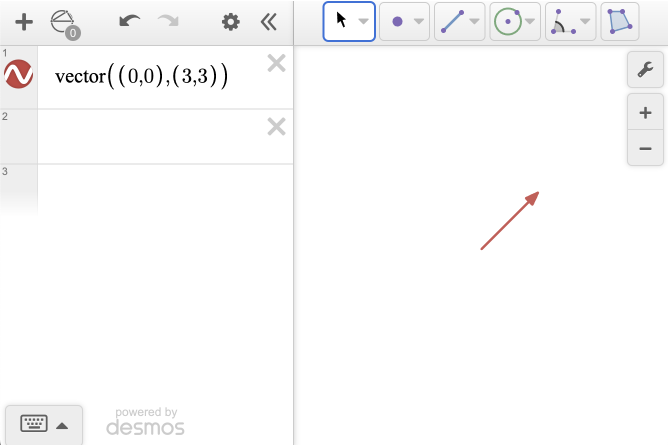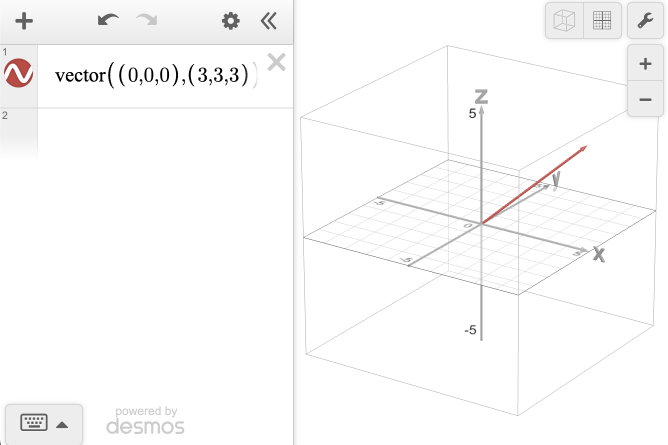# Vectors and Point Operations

Desmos Calculators allow you to visualize vector operations on points. In Desmos 3D and the Geometry tool, you can graph vectors by typing $$vector(a,b)$$ with starting point $$a$$ and terminal point $$b$$. For example, $$vector((0,0,0),(3,3,3))$$ will graph a 3D vector in standard position with terminal point $$(3,3,3)$$.### Operations on Points and Vectors

You can also perform operations with points and vectors! Point operations work in the Graphing Calculator, Geometry Tool, and 3D Calculator. Vector operations are currently only supported in the Geometry Tool and 3D Calculator.

In the table below, $$a$$ and $$b$$ represent points and $$v_1$$ and $$v_2$$ represent vectors. You can explore these operations in this example graph.

Function: Result:
$$a.x, a.y, a.z$$ Outputs the x, y, and z component of your point (numerical).
$$v_1$$.start Plots the initial point of your vector.
$$v_2$$.end Plots the terminal point of your vector.
$$|a|$$ or $$|v|$$ Outputs the magnitude of your vector. On a point, it outputs the magnitude of the vector in standard position to point a (numerical).
$$a + b$$ or $$v_1+v_2$$ Graphs the vector sum of two points or vectors.
$$a - b$$ or $$v_1-v_2$$ Graphs the vector difference of two points or vectors.
$$ka$$ or $$kv$$ Graphs the scalar multiple of a constant, k, times a point or vector.
$$v_1$$$$\cdot$$$$v_2$$ Outputs the scalar value that results when you multiply two vectors. This is found by multiplying the x components, y components, and z components (in 3D) and then summing the results (numerical).
$$v_1$$$$\times$$$$v_2$$ Graphs the vector perpendicular to the plane containing the original 2 vectors. Note: Cross product is only defined in 3D. To get the symbol $$\times$$, type the word ‘cross.’